Publicité

# Decision tree

16 Apr 2020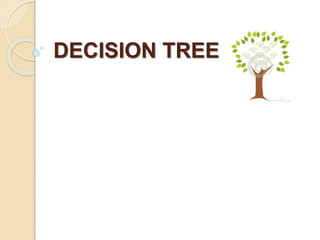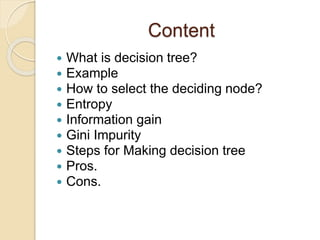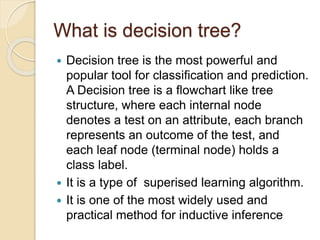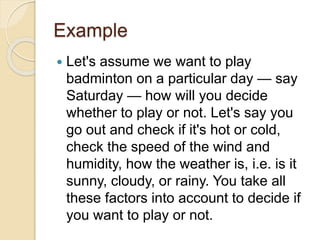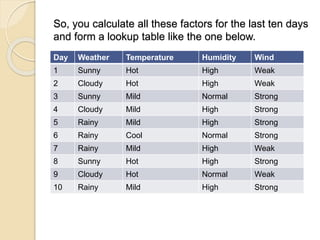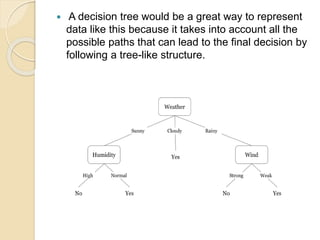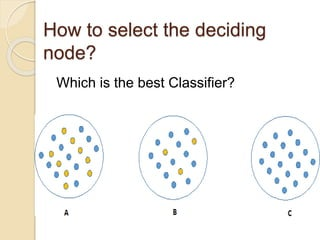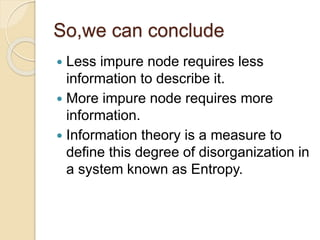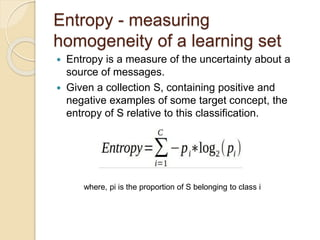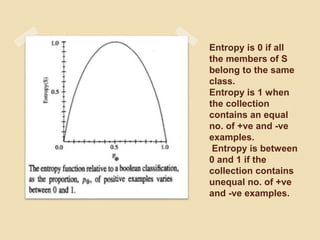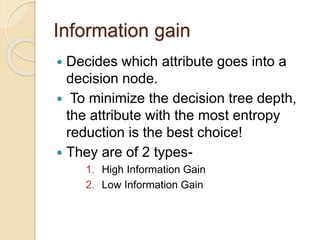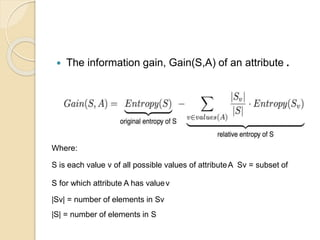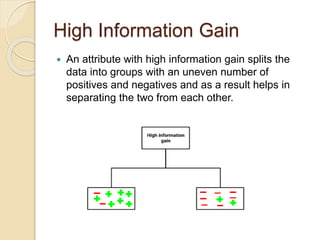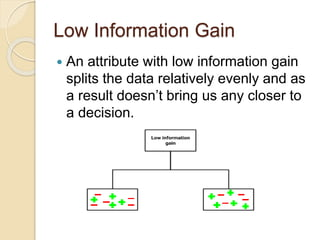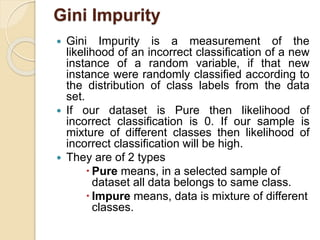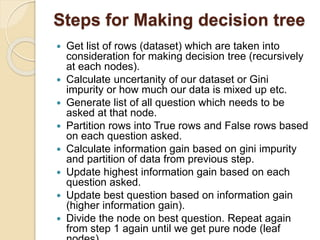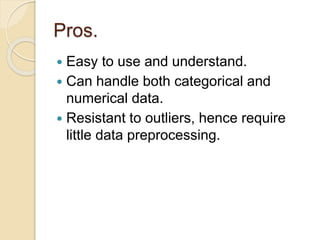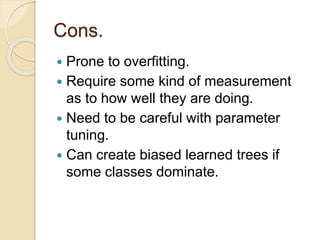1 sur 19
Publicité

### Decision tree

1. DECISION TREE
2. Content  What is decision tree?  Example  How to select the deciding node?  Entropy  Information gain  Gini Impurity  Steps for Making decision tree  Pros.  Cons.
3. What is decision tree?  Decision tree is the most powerful and popular tool for classification and prediction. A Decision tree is a flowchart like tree structure, where each internal node denotes a test on an attribute, each branch represents an outcome of the test, and each leaf node (terminal node) holds a class label.  It is a type of superised learning algorithm.  It is one of the most widely used and practical method for inductive inference
4. Example  Let's assume we want to play badminton on a particular day — say Saturday — how will you decide whether to play or not. Let's say you go out and check if it's hot or cold, check the speed of the wind and humidity, how the weather is, i.e. is it sunny, cloudy, or rainy. You take all these factors into account to decide if you want to play or not.
5. So, you calculate all these factors for the last ten days and form a lookup table like the one below. Day Weather Temperature Humidity Wind 1 Sunny Hot High Weak 2 Cloudy Hot High Weak 3 Sunny Mild Normal Strong 4 Cloudy Mild High Strong 5 Rainy Mild High Strong 6 Rainy Cool Normal Strong 7 Rainy Mild High Weak 8 Sunny Hot High Strong 9 Cloudy Hot Normal Weak 10 Rainy Mild High Strong
6.  A decision tree would be a great way to represent data like this because it takes into account all the possible paths that can lead to the final decision by following a tree-like structure.
7. How to select the deciding node? Which is the best Classifier?
8. So,we can conclude  Less impure node requires less information to describe it.  More impure node requires more information.  Information theory is a measure to define this degree of disorganization in a system known as Entropy.
9. Entropy - measuring homogeneity of a learning set  Entropy is a measure of the uncertainty about a source of messages.  Given a collection S, containing positive and negative examples of some target concept, the entropy of S relative to this classification. where, pi is the proportion of S belonging to class i
10. Entropy is 0 if all the members of S belong to the same class. Entropy is 1 when the collection contains an equal no. of +ve and -ve examples. Entropy is between 0 and 1 if the collection contains unequal no. of +ve and -ve examples.
11. Information gain  Decides which attribute goes into a decision node.  To minimize the decision tree depth, the attribute with the most entropy reduction is the best choice!  They are of 2 types- 1. High Information Gain 2. Low Information Gain
12.  The information gain, Gain(S,A) of an attribute . Where: S is each value v of all possible values of attributeA Sv = subset of S for which attribute A has valuev |Sv| = number of elements in Sv |S| = number of elements in S
13. High Information Gain  An attribute with high information gain splits the data into groups with an uneven number of positives and negatives and as a result helps in separating the two from each other.
14. Low Information Gain  An attribute with low information gain splits the data relatively evenly and as a result doesn’t bring us any closer to a decision.
15. Gini Impurity  Gini Impurity is a measurement of the likelihood of an incorrect classification of a new instance of a random variable, if that new instance were randomly classified according to the distribution of class labels from the data set.  If our dataset is Pure then likelihood of incorrect classification is 0. If our sample is mixture of different classes then likelihood of incorrect classification will be high.  They are of 2 types  Pure means, in a selected sample of dataset all data belongs to same class.  Impure means, data is mixture of different classes.
16. Steps for Making decision tree  Get list of rows (dataset) which are taken into consideration for making decision tree (recursively at each nodes).  Calculate uncertanity of our dataset or Gini impurity or how much our data is mixed up etc.  Generate list of all question which needs to be asked at that node.  Partition rows into True rows and False rows based on each question asked.  Calculate information gain based on gini impurity and partition of data from previous step.  Update highest information gain based on each question asked.  Update best question based on information gain (higher information gain).  Divide the node on best question. Repeat again from step 1 again until we get pure node (leaf
17. Pros.  Easy to use and understand.  Can handle both categorical and numerical data.  Resistant to outliers, hence require little data preprocessing.
18. Cons.  Prone to overfitting.  Require some kind of measurement as to how well they are doing.  Need to be careful with parameter tuning.  Can create biased learned trees if some classes dominate.
Publicité